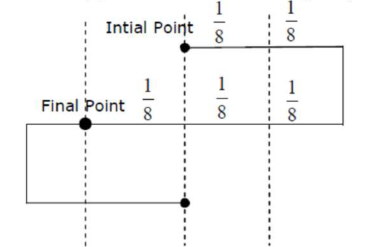# Time period of a simple pendulum is T.Question:

Time period of a simple pendulum is $T$. The time taken to complete $\frac{5}{8}$ oscillations starting from mean position is $\frac{\alpha}{\beta} T$. The value of $\alpha$ is

Solution:

(7)

For given $\left(\frac{5}{8}\right)$ oscillation, we can write it as $\rightarrow\left(\frac{1}{2}+\frac{1}{8}\right)$ And we know for half oscillations time $\rightarrow \frac{T}{2}$For final point $\rightarrow \pi+\frac{\pi}{6} \quad \Rightarrow \frac{7 \pi}{6}$

Time $\rightarrow \frac{7 T}{12} \rightarrow$ given $\rightarrow \frac{\alpha}{\beta} T \alpha=7 \mathrm{p}$